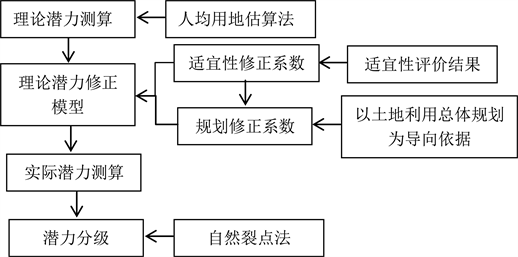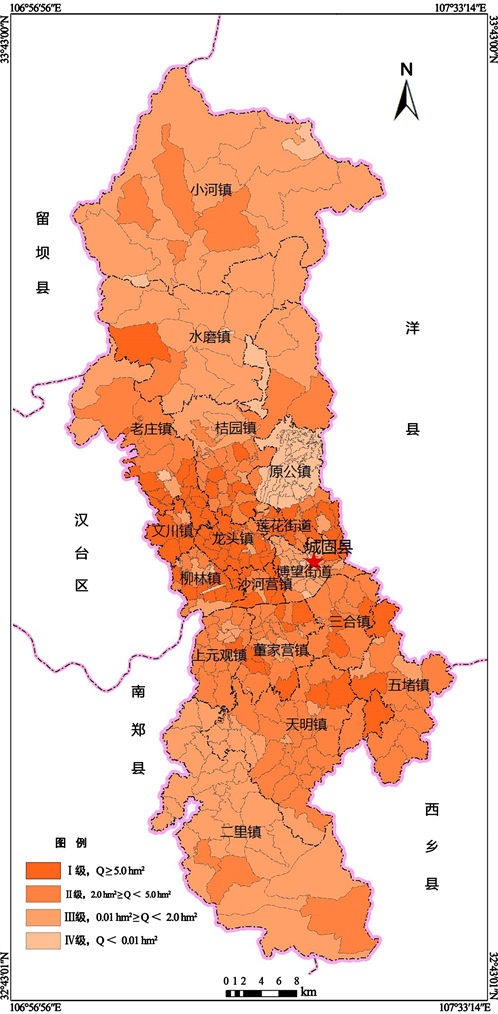# 农村建设用地整理潜力测算与分级研究A Study on the Measurement and Classification of Rural Construction Land Consolidation Potential

• 全文下载: PDF(3393KB)    PP.77-82   DOI: 10.12677/ULU.2018.64010
• 下载量: 318  浏览量: 1,103   国家科技经费支持

To quantify scientifically rural construction land consolidation, the correction model is established based on the per capita estimation method. The model can correct the theoretical potential for suitability and planning correction. Natural split point method is used to classify potential. The results show that the modified rural construction land consolidation potential is closer to the actual, more scientific and reasonable and the potential classification by natural split point method is more reasonable and can provide a scientific guidance to rural construction land consolidation direction. The results can be used for the preparation of land remediation planning, and provide the basis for the promotion and implementation of rural construction land consolidation activities smoothly.

1. 引言

2. 研究思路与数据来源

1) 研究思路Figure 1. The technology frame

2) 数据来源

3. 实证研究

1) 研究区概况

2) 理论潜力的测算

${Q}_{t}={S}_{0}-{B}_{t}×{P}_{t}={S}_{0}-{B}_{t}×\left({P}_{0}×{\left(1+r\right)}^{n}±\Delta P\right)$ (1)

3) 适宜性修正系数测算

$f\left(Y\right)=\underset{i=1}{\overset{n}{\sum }}\left(\frac{{S}_{i}}{S}×n{Y}_{i}\right)$ (2)

4) 规划修正系数测算

$f\left(G\right)=\left(\underset{i=1}{\overset{n}{\sum }}{S}^{{G}_{g=1}}i\right)/S$ (3)

5) 测算农村建设用地整理实际潜力

$Q={Q}_{t}×f\left(Y\right)×f\left(G\right)$ (4)

6) 农村建设用地整理潜力分级Table 1. Rural construction land rearrangement potential classification of ChengguFigure 2. Rural construction land rearrangement potential classification of Chenggu

4. 结果分析

5. 结论与讨论

  李宪文, 张军连, 郑伟元, 等. 中国城镇化过程中村庄土地整理潜力估算[J]. 农业工程学报, 2004, 20(4): 276-279.  刘晓燕, 刘峰, 夏英晓, 等. 农村居民点土地整理潜力测算方法研究——以烟台市牟平区为例[J]. 山东国土资源, 2011, 27(2): 35-37.  王雨桐, 李钢, 赵华, 等. 村域尺度农村居民点整理潜力测算研究[J]. 湖北农业科学, 2014, 56(9): 2197-2202.  宋伟, 张凤荣, 陈曦炜. 我国农村居民点整理潜力测算方法研究[J]. 广东土地科学, 2006(5): 43-46.  石诗源, 张小林. 江苏省农村居民点用地现状分析与整理潜力测算[J]. 中国土地科学, 2009, 23(9): 52-58.  陈荣清, 张凤荣, 孟媛, 等. 农村居民点整理的现实潜力估算[J]. 农业工程学报, 2009, 25(4): 216-222.  许晓婷. 县级土地整治规划理论与方法研究[D]. 西安: 长安大学, 2014.  曲衍波, 张凤荣, 宋伟, 等. 农村居民点整理潜力综合修正与测算——以北京市平谷区为例[J]. 地理学报, 2012, 67(4): 490-503.  宋伟, 陈百明, 姜广辉. 中国农村居民点整理潜力研究综述[J]. 经济地理, 2010, 30(11): 1871-1877.